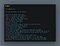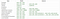# Learning The Pythonic Way

## Some cool features and tips to write more Pythonic codePhoto by Tudor Baciu on Unsplash

While I am still learning to get better at Python every day, I wanted to share some of the resources that have helped me in my learning process and I hope this article will guide you in exploring more features of this language.

As this article states, when a veteran Python developer (a Pythonista) calls portions of code not Pythonic, they usually mean that these lines of code do not follow the common guidelines and fail to express its intent in what is considered the best (hear: most readable) way.

Tim Peters, the Core Developer and author of The Zen of Python, said:

The core tenents of writing good Python code as he states are:The Zen of Python

## Here are a few practices and features that will help you write idiomatic and Pythonic code:

• Naming Conventions: The PEP8 (Python Enhancement Proposal) guidelines suggest some specific naming conventions such as:Naming Conventions in Python
• Multiple Assignment and Iterable Unpacking: Python supports assigning the same values to multiple variables. For example:
`# assigning a and b as 100a = b = 100`

We can unpack elements of iterable (tuple, list, string, etc.) as shown below:

`# Iterable UnpackingX Not recommendedemployees = ["Carlos", "Badal", "Manoj"]first = employeessecond = employeesthird = employees=> Recommendedfirst, second, third = employeesXX ValueError: too many values to unpack if : first, second, third = ["Carlos", "Badal", "Manoj", "Michael"] XX ValueError: not enough values to unpack if :first, second, third = ["Carlos", "Badal"]# Unpacking generatorsresult = (i ** 3 for i in range(5))zero, one, two, three, four = result# Unpacking a dictionarydicts = {'one': 1, 'two':2, 'three': 3, ‘four’:4} * This will unpack keysa, b, c, d = dicts* This will unpack valuesa, b, c, d = dicts.values() * This will unpack (key, value) pair a, b, c, d = dicts.items()# Using * operator a, *b = 1, 2, 3 => a = 1     => b = [2,3]first, *second, third = [1,2,3,4,5]=> first = 1  => second = [2,3,4]  => third = 5 # Swapping valuesX Not recommendedtemp = a a = b b = temp=> Recommendeda, b = b, aVery useful in sorting tasks while doing: a[i-1], a[i] = a[i], a[i-1]`
• Comparison: To avoid confusion in identity and equality comparison, you should be clear on ‘is’ (identity comparison) and ‘==’ (value comparison). In Python, ‘is’ returns true if two references refer to the same object and ‘==’ returns true if two objects have the same value.
`a = [1, 2, 3, 4]b = a=> b is areturns True=> b == a returns TrueBut if we make a new copy of a and compare:b = a[:]=> b is areturns False=> b == a returns True`
• Loops: Using methods such as enumerate and zip with loops, we can simplify our program.
`# Enumerate Allows you to keep track of index along with variable while looping throuh an iterable:employees = [‘Carlos’,’Badal’,’Michael’]X Not recommendedfor idx in range(len(employees)):     print(f'{idx}:{employees[idx]}')=> Recommendedfor idx, name in enumerate(employees):     print(f'{idx}:{name}')# ZipAllows you to easily work with multiple lists of related data:employees = [‘Carlos’,’Badal’,’Michael’]age_records = [25, 26, 27]for name, age in zip(employees, age_records):   print(f"{name} is {age}.")`
• List Comprehension: This is one of my favorite features in Python for its elegance and readability. It is much easier to understand for beginners and also performs faster than its alternatives most of the time.

From the documentation:

Some examples:

`X Not Recommendedresult = []for i in range(5):    result.append(i**3)=> Recommendedresult = [i**3 for i in range(5)]* We can do so much moredicts = {‘james’: '100', 'donna’: '200', 'smith': '50'}result = [dicts[x] for x in dicts]a = [1, 2, 3] b = [4, 5, 6] result = [x - y for x in a for y in b]# Nested List Comprehensiona = [[1,2],[3,4],[5,6]]b = [x for each in a for x in each]=> b will be [1, 2, 3, 4, 5, 6]`

Tip: Always consider the readability of your code before implementing list comprehension and avoid making it complex.

• Avoid Map, Filter & Reduce (whenever possible): Map, Filter & Reduce functions bring a bit of functional programming to Python providing a short-hand approach to some problems and can be replaced with list comprehension and loops.

In this article, Guido describes how using list comprehension, loops and other methods might make your code clearer and readable for others.

I definitely use these features myself for some specific tasks but it is recommended to use list comprehension or generator expressions (instead of map & filter) and loops (instead of reduce) when possible since they serve a similar purpose and are considered more Pythonic.

Examples of map() and filter():

`# map()Map is still very useful when performing a task like this:map(str, range(100))instead of:[str(item) for item in range(100)]But :=> Recommended: List Comprehension[expression(item) for item in iterable]or, (expression(item) for item in iterable) -> generator expressionsX Not Recommendedmap(lambda item: expression(item), iterable)# filter():def some_function(x):     return x % 2 != 0 and x % 3 != 0X Not Recommendedresult = list(filter(some_function, range(2, 25)))=> Recommened: List Comprehensionresult = [i for i in range(2,25) if some_function(i)]X Not Recommendedlist(map(lambda x:x+1, filter(lambda x:x%3, range(10))))=> Recommended: List Comprehension[x+1 for x in range(10) if x%3]`

reduce() has been dropped from the built-in functions and can be used from functools module.

Guido states in the article:

Example of reduce():

`my_list = [10,15,20,25,35]X Not Recommendedfrom functools import reducetotal_sum = reduce(lambda x ,y : x+y , my_list)=> Recommendedtotal_sum = 0for num in my_list:    total_sum += numor, total_sum = sum(my_list)`

These features definitely have more use cases and in the end, it really depends on your preference.

# Conclusion

I hope this article has helped you understand and learn some conventions used in Python. There are tons of great resources online and several StackOverflow discussions you can look into.

Happy Coding!

Software Engineer Intern. ML Enthusiast.

## More from Manoj Aryal

Software Engineer Intern. ML Enthusiast.